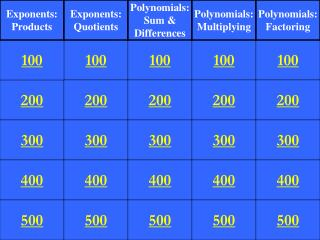DownloadDownload Presentation200

# 200

Télécharger la présentation## 200

- - - - - - - - - - - - - - - - - - - - - - - - - - - E N D - - - - - - - - - - - - - - - - - - - - - - - - - - -
##### Presentation Transcript

1. Exponents: Products Exponents: Quotients Polynomials: Sum & Differences Polynomials: Multiplying Polynomials: Factoring 100 100 100 100 100 200 200 200 200 200 300 300 300 300 300 400 400 400 400 400 500 500 500 500 500

2. Simplify

3. Simplify:

4. For all real numbers m and n, which of the following statements is always true?

5. B

6. The length of a rectangle isThe width of the rectangle isWhat is the area in simplified form?

7. Prove or disprove the following statement: (xy)2 = xy2

9. Simplify

10. Simplify:

11. Simplify

12. The area of a rectangle is:The width is:What is the length?

13. Simplify

14. Simplify

15. Simplify

16. Simplify

17. Darnell and Stephanie have competing refreshment stand businesses. Darnell’s profit can be modeled with the polynomial , where c is the number of items sold. Stephanie’s profit can be modeled with the polynomial . • Write a polynomial that represents • the difference between Stephanie’s • profit and Darnell’s profit.

18. Simplify

19. Simplify

20. Simplify

21. Simplify

22. Simplify(x2+y)(x+y+z)

23. What is the greatest common factor of…

24. Factor the polynomial using the GCF.

25. Factor the polynomial into a product of two binomials.

26. Factor the polynomial into a product of two binomials.

27. Factor the polynomial into a product of two binomials.